×

Search anything:

# Understand basic TensorFlow programming concepts

#### Machine Learning (ML) TensorFlowGet this book -> Problems on Array: For Interviews and Competitive Programming

TensorFlow is one of the most efficient Machine Learning framework available today and has widely adoption globally. It is used to power several production level applications like Google Search, Photo, Map, Translate, Gmail and others. We will take a look at the basic programming principles of TensorFlow by building a very simple model to evaluate an arithmetic expression.

### TensorFlow classes

TensorFlow follows an Object Oriented Programming design and hence, we have classes using which we create objects to build our Machine Learning model. There are three basic TensorFlow classes:

• Graph
• Operation
• Tensor

### Graph

A TensorFlow is a collection of Operations and Tensors.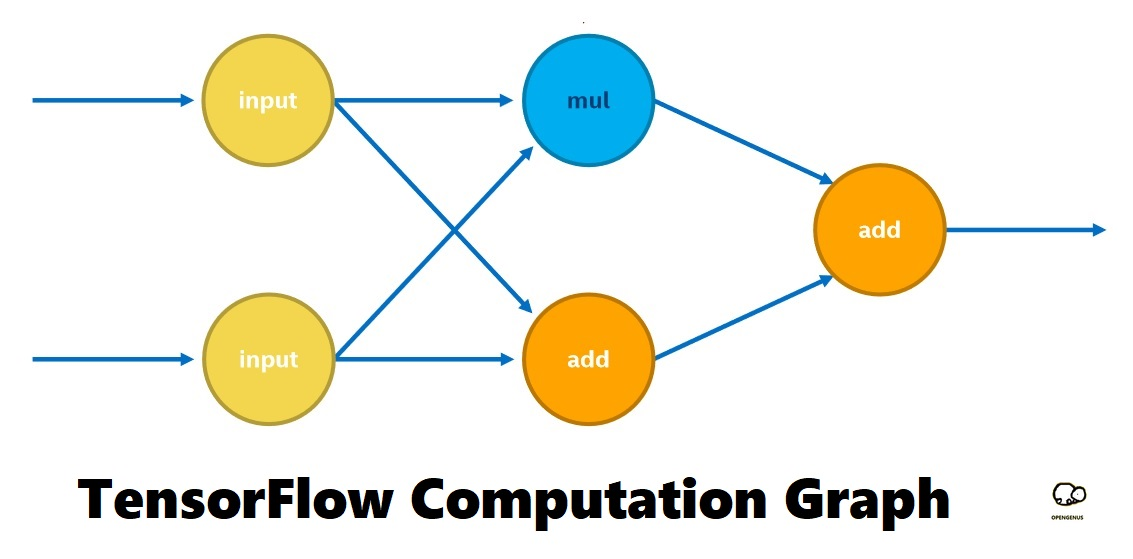### Operation

Operations are nodes in the TensorFlow Computational Graph and it represents mathematical operations to be computed. The number of inputs and outputs depends on the operation represented by the operation node.

Some of the common operation nodes are:

• Multiplication
• Matrix Multiplication
• Convolution

and others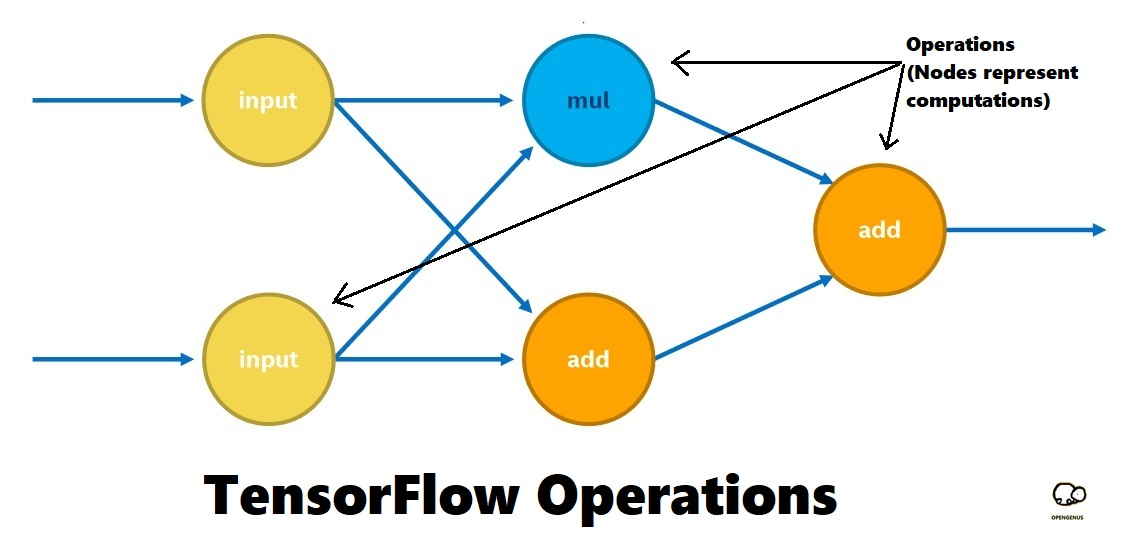### Tensor

Tensors are the edges in the TensorFlow Computational Graph and represent data flow. The data can be of any form and is determined by the associated operations (nodes). Common tensors are:

• 1 X 1 data (integers, float (16, 32, 64 bit)
• N x N data (matrix)
• N x M x 3 data (non-transparent 3 bit images)

and many others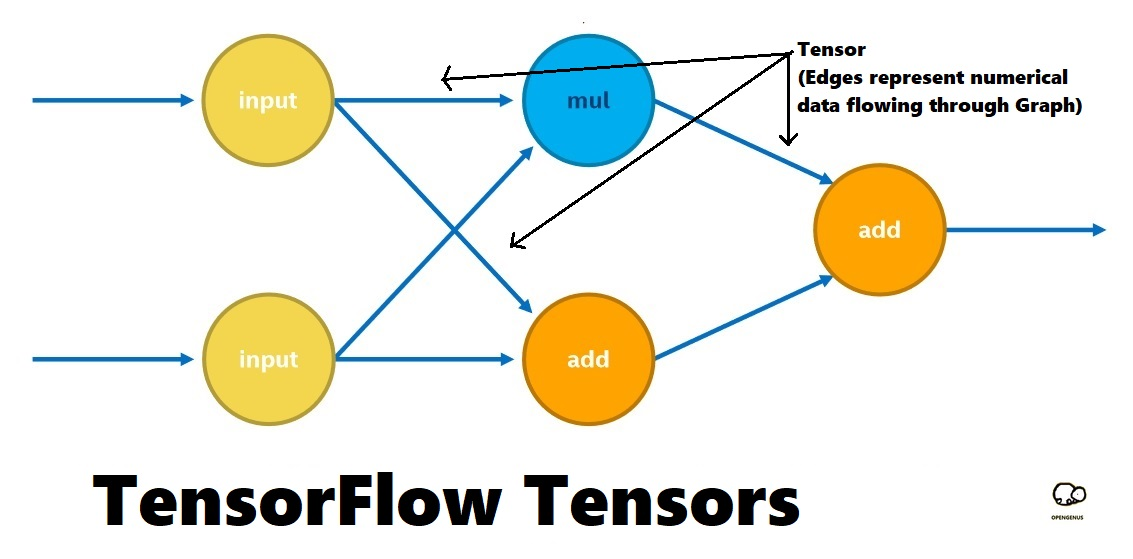### Data flow example

Following example illustrates the TensorFlow approach to compute the expression:

((X x Y) + (X + Y))

where X = 7 and Y = 3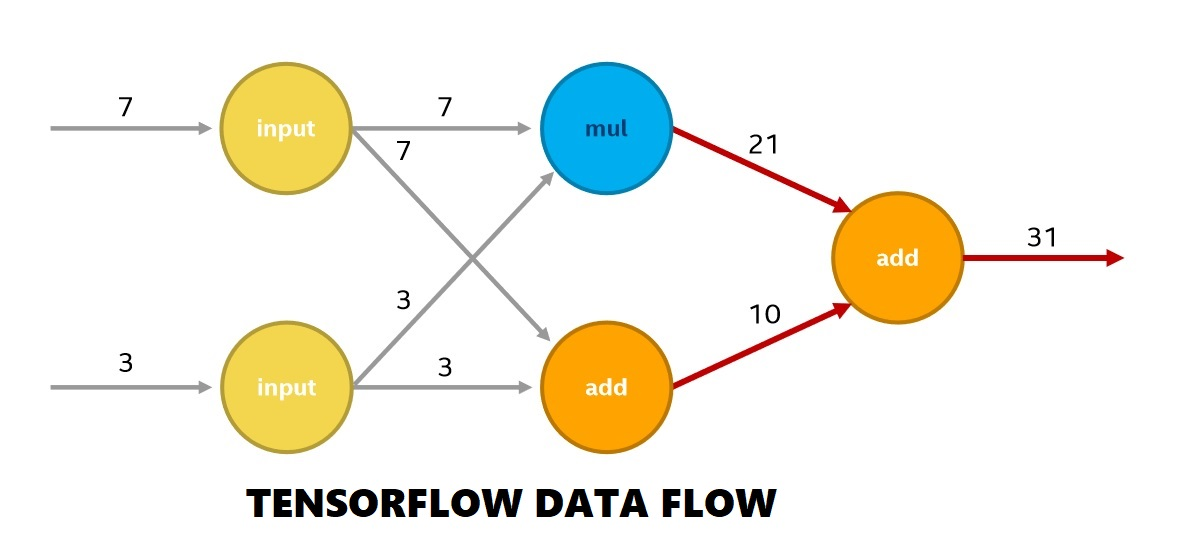#### Create a TensorFlow node (operation)

To do this, you need to understand two basic TensorFlow functions:

• tf.constant() creates an Operation that returns a fixed value

There are several other operations that we will cover in another article

#### Create an input data

To define an user defined data, use:

• tf.placeholder() defines an user defined input that vary run to run

In general Machine Learning applications, we have a process known as training which generates some of the input parameters such as weights and bias to be used. For it, we need to use tf.Variable()

### Code Example

Consider this code fragment:

``````
# Create a placeholder data a
a = tf.placeholder(tf.float32, name="input1")
# Create a placeholder data b
b = tf.placeholder(tf.float32, name="input2")
# Create an add operation using a and b
``````

In this code, we are adding two inputs a and b and storing it in c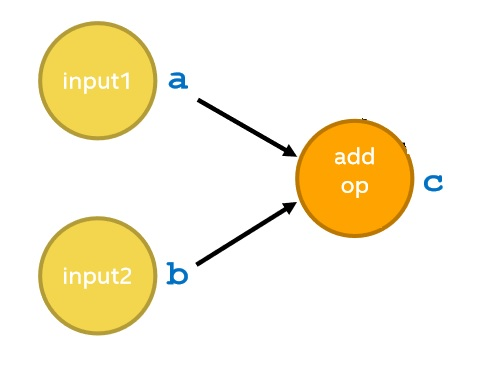### Create a TensorFlow session

A TensorFlow session is like a runtime or a container where the program will execute. To create a session, use:

``````
sess = tf.Session()
``````

A session has several properties and to set it, we need ConfigProto. It is used to set configurations of the Session object.

This code creates a session and sets two custom configuration values:

``````
tf.Session(config=config)
``````

### Pass input using placeholder

We have created our placeholders and now, we will give them values through a dictionary data structure which will be passed to session.

``````
feed_dict = {a: 3.0, b: 2.0}
``````

### Execute the graph

To execute the graph, use:

``````
sess.run(graph, feed_dict)
``````

sess.run returns the fetched values as a NumPy array

The complete code to add two numbers in TensorFlow is:

``````
import tensorflow as tf
# Create a placeholder data a
a = tf.placeholder(tf.float32, name="input1")
# Create a placeholder data b
b = tf.placeholder(tf.float32, name="input2")
# Create an add operation using a and b
sess = tf.Session(config=config)
feed_dict = {a: 3.0, b: 2.0}
output = sess.run(c, feed_dict)
print (output)
``````

### Enjoy

You know have the basic idea of how to perform basic arithmetic operations in TensorFlow.